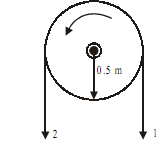## Calculate tension in limbs, Mechanical Engineering

Assignment Help:

Calculate tension in limbs:

Q : A horizontal drum of belt drive carries the belt over semicircle around it. It is rotated counter clockwise to transmit a torque of 300N-m. If coefficient of friction between the belt and rope is 0.3, calculate tension in limbs 1 and 2 of belt shown in figure, and reaction on bearing. The drum is having mass of 20Kg and the belt is assumed to be mass less.Sol: Given: Torque(t) = 300N-m

Coff. of friction(µ) = 0.3

Diameter of Drum (D) = 1m, R = 0.5m

Mass of drum(m) = 20Kg.

Since angle of contact = p rad Torque = (T1  - T2).R

300 = (T1  - T2) X 0.5

T1- T2  = 600N                                                                                                                              ...(i)

And,     T1/T2  = eµθ

T1/T2  = e(0.3)p

T= 2.566T2                                                                                                                               ...(ii)

Solve (i) and (ii)

We get,

T1  = 983.14N                                                                .......ANS

T2  = 383.14N   .......ANS

Now reaction on bearing is opposite to mass of body, and it is equal to

R = T1  + T2  + mg

R = 983.14 + 383.14 + 20 X 9.81

R = 1562.484N                                                            .......ANS

#### Revited joints, which material is used in production of solid revits

which material is used in production of solid revits

#### Elevator, These are differentiated from hoists by the fact that the opera...

These are differentiated from hoists by the fact that the operator rides with the load. There are many different types of drives for such elevators but in general electric

#### Location of centre of gravity of a hemisphere, Location of centre of gravit...

Location of centre of gravity of a hemisphere: Find out the location of centre of gravity of a hemisphere of radius a. Solution Figure shows the cross-section of the h

#### Magnesium and its alloys, Magnesium And Its Alloys Magnesium is a light...

Magnesium And Its Alloys Magnesium is a light metal along with density of 1.74 g/cm 3 . Magnesium is more costly than aluminium as density 2.74 g/cm 3 along with which it comp

#### Explain point angle - angle of drills, Explain POINT ANGLE - Angle of drill...

Explain POINT ANGLE - Angle of drills It is also known as a cutting angle. Its most commonly used value for a large variety of materials is 118.Though,its varies from 80 to 140

#### Dual combustion cycle, In an I.C. engine operating on the Dual combustion c...

In an I.C. engine operating on the Dual combustion cycle, the temperature of the working fluid (air) at the beginning of compression is 27ºC. The ratio of the maximum and minimum p

#### Overbridges - fly-overs and underpasses, Overbridges, Fly-Overs And Underpa...

Overbridges, Fly-Overs And Underpasses: Railway Over-bridges Railway Over-bridges (ROB) are structures constructed to enable the road traffic to go over the railway t

#### Strenght of material, how to calculate point of contraflexure in SFD and BM...

how to calculate point of contraflexure in SFD and BMD

#### Renewable Energy, I am attending Thermodynamics class at the university. Th...

I am attending Thermodynamics class at the university. The professor asked me to write a research on Renewable Energy: Solar Power. The report should be of 10 pages and at least 10

#### Cantilever beam stress, Dear Engineer! I have an aluminum tube(6061) fixed...

Dear Engineer! I have an aluminum tube(6061) fixed at one end (Cantilever Beam). The length is 180” and the load on the other end is 150 lbs. Question: What size of tubes I s